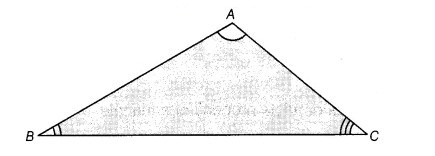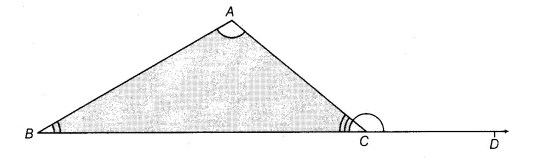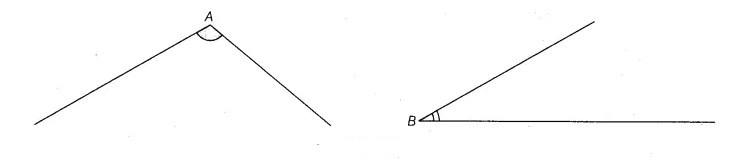# Objective: To verify exterior angle property of a triangle

Lets verify exterior angle property of a triangle. Class 9 Maths lab manual experiment and activities to conduct.

## Materials Required

1. Cardboard sheet
2. Glazed papers
3. Geometry box
4. White chart paper
5. Tracing paper
7. Cutter

### Prerequisite Knowledge

Before verifying the exterior angle property of a triangle pleas go through the topics of Straight angle and Exterior angle property of a triangle

### Theory

For straight line refer to Activity 11.

For exterior angle property of a triangle refer to Activity 12.

### Procedure

1. Take a cardboard sheet of suitable size and by using adhesive, paste a white chart paper on it.

2. Cut out a ΔABC from a glazed paper and paste it on the cardboard as shown in the image below:3. Now, produce the side BC of ΔABC to the point D; refer figure below4. Cut out the pair of angles, ∠A and ∠B from the glazed paper by using a tracing paper, as shown below5. Now, arrange the cut out angles as shown in the below image### Demonstration

From the last figure above,

∠ACD is an exterior angle.
∠A and ∠B are its two interior opposite angles.
The two cut out angles, ∠A and ∠B together completely cover ∠ACD. So, ∠ACD = ∠A + ∠B.

### Observation

Measure of ∠A = ........., and ∠B = ............, Sum of (∠A + ∠B) = ..........., and measure of ∠ACD = ..........., Therefore, ∠ACD = ∠A + ∠B.

### Result

We have verified the exterior angle property of a triangle.

### Application

Many geometrical problems may be easily done, using this property of a triangle.

### Viva Voce

Question 1: What do you mean by the linear pair axiom?
Answer: If a ray stands on a line, then the sum of the two adjacent angles so formed is 180° and vice-versa. This property is known as linear pair axiom.

Question 2: What would be the measure of exterior angle for each vertex of equilateral triangle?

Question 3: Does an exterior angle of a triangle is smaller than either of its interior opposite angles?
Answer: No, an exterior angle of a triangle is greater than either of its opposite interior angles.

Question 4: If the exterior angle of a triangle is 90o, then what would be the opposite interior angles, supplementary or complementary?

Question 5: How would you define an exterior angle property of a triangle?
Answer: An exterior angle of a triangle is equal to sum of opposite interior angles.

Question 6: If the sum of interior opposite angles of a triangle is 130o, then what would be the measure of its exterior angle?
Answer: Sum of interior opposite angles = Exterior angle Exterior angle = 130o.

Question 7: What is the angle form by straight line?
Answer: The straight line always form a angle of 180o.

Question 8: What is the condition of exterior angle of a triangle?
Answer: The exterior angle of a triangle is equal to the sum of opposite interior angles.

Question 9: What is the sum of exterior angles of a triangle?
Answer: We know that the sum of exterior angles of a polygon is always 360°. The sum of exterior angles of a triangle is 360o.

### Latest Articles### Spreading Knowledge Across the World

USA - United States of America  Canada  United Kingdom  Australia  New Zealand  South America  Brazil  Portugal  Netherland  South Africa  Ethiopia  Zambia  Singapore  Malaysia  India  China  UAE - Saudi Arabia  Qatar  Oman  Kuwait  Bahrain  Dubai  Israil  England  Scotland  Norway  Ireland  Denmark  France  Spain  Poland  and  many more....